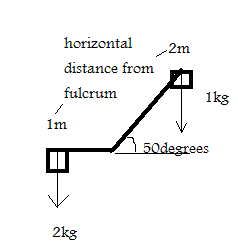# Lever question when lever is angled

• JCaleb

#### JCaleb

Hello, I have a question about a see-saw / lever problem. Does this configuration I have shown here allow me to treat the system like a regular lever if I make the downward force at the horizontal point from the center to be the cosine of the angle? In this example the downward force on the left would be 2kg at 1m whereas on the right it would be 1kg * cos(5) at 2m.

Based on the static equilibrium equation of a lever F1*d1= F2*d2 the system would not be in equilibrium and would fall to the left. 2*1 > 0.64*2

Is this correct?

Would that mean that there is a compression force of the sine of the angle down the shaft towards the fulcrum on the right side? 1kg*sin(50) ?#### Attachments

In this example the downward force on the left would be 2kg at 1m whereas on the right it would be 1kg * cos(5) at 2m.

How do you define the distance?

Based on the static equilibrium equation of a lever F1*d1= F2*d2 the system would not be in equilibrium and would fall to the left. 2*1 > 0.64*2p

The object will fall unless a 1m point is made to hold. If this is done, then it'll be a net equilibrium.

No it will not rotate while falling due to gravity.